Example Questions

Example Question #1 : Squares

In the figure above, the circle is inscribed within the bounding square. If r = 5, what is the area of the shaded region?

25 - (25/4)π

25π

100

100π

100 - 25π

25 - (25/4)π

Explanation:

In order to solve this, we must first find the area of the containing square and then remove the inscribed circle.  Once this is done, we need to divide our result by 4 in order to get the one-forth that is the one shaded region.

One side of the square will be equal to the circle's diameter (2r).  Since r = 5, d = 10.  Therefore, the area of the square is d2 = 102 = 100.  The area of the circle is πr2 = 52π = 25π.

Therefore, the area of the four "corner regions" is equal to 100 - 25π.  One of these is equal to (100 - 25π) / 4.  Simplified, this is 25 - (25/4)π.

Example Question #1361 : Gre Quantitative Reasoning

Find the area of a square with a side length of 4.

32

24

16

8

16

Explanation:

All sides are equal in a square. To find the area of a square, multiply length times width. We know length = 4 but since all sides are equal, the width is also 4. 4 * 4 = 16.

Example Question #3 : How To Find The Area Of A Square

If one doubles the radius of the semi-circle on the right of the diagram above, by what percentage does the overall area of the diagram change?

400%

200%

150%

100%

300%

300%

Explanation:

To compare, first calculate the area of figure 1.  Since it shares dimensions with the semi-circle, we will put all our variables in terms of the radius of that semi-circle:

A1 = (2r)2 + πr2/2 = 4r2 + πr2/2 = r2(4 + π)/2

If we double r, we get:

A2 = (2 * 2r)2 + π(2r)2/2 = 16r2 + π4r2/2 = 4r2(4 + π)/2

This means that the new figure is 4x the size of the original.  This is an increase of 300%.

Example Question #1 : How To Find The Area Of A Square

100 cm = 1 m

Quantity A: The area of a square with side 1 m

Quantity B: One hundred times the area of a square with side 1 cm

Quantity A is greater.

The two quantities are equal.

Quantity B is greater.

The relationship cannot be determined from the information given.

Quantity A is greater.

Explanation:

The obvious answer for this problem is that they are equal.  Remember quantitative comparisons are often tricky and require you to check your initial inclination. If 1 m equals 100 cm then a square with side 1 m (100 cm) has an area of 100 cm x 100 cm or 10,000 cm2. The area of a square with a 1 cm side is 1 cm x 1 cm or 1 cm2. One hundred times 1 cmis 100 cm2. 10,000 cm2 is larger than 100 cm2 so Quantity A is greater.

Example Question #2 : Squares

A square is inscribed in a circle. The diameter of the circle is 10 feet. What is the area of the square?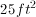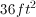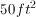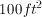It cannot be determined from the information provided.Explanation:

Since the diameter of the circle is 10, we know the radius of the circle is 5 feet. We can then draw radii that go from the center to two consecutive corners of the square. These radii are both 5 feet, and form a 90 degree angle (since they are diagonals of a square). Thus, with the enclosed side of the square they form a 45-45-90 triangle. Thus, the side of the square must be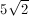(this can also be found with the Pythagorean theorem). Then, the area of the square is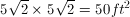Example Question #6 : How To Find The Area Of A Square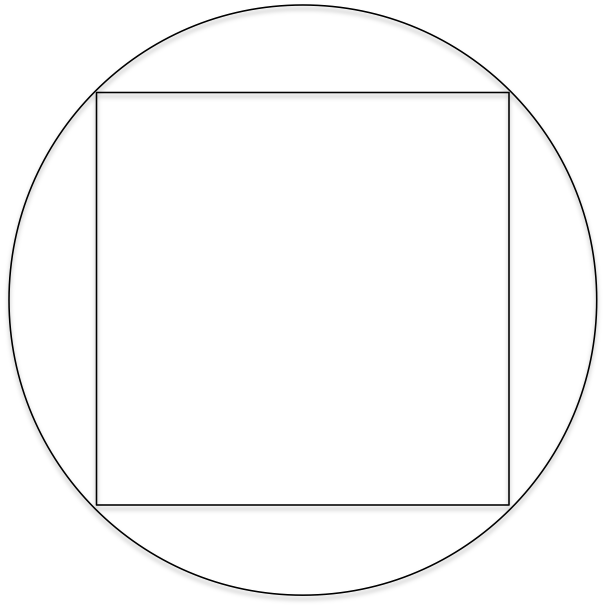In the figure above, a square is inscribed in a circle with a diameter of 5 cm.

What is the area of the square?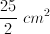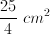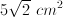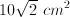Explanation:

The diameter of the circle and the sides of the square form a 45-45-90 triangle. Since the hypotenuse is 5 cm, then a leg of the triangle (a side of the square) is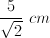.

The area of the square is then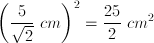.

Example Question #1 : How To Find The Area Of A Square

Square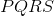is on a coordinate plane, and each side of the square is parallel to either the-axis or-axis. Pointhas the coordinate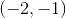and Pointhas the coordinate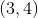.

Quantity A: The area of squareQuantity B: 24

Quantity A is greater

The two quantities are equal

The information cannot be determined based on the information provided

Quantity B is greater

Quantity A is greater

Explanation:

If you draw pointsandon the coordinate plane, you know that they are opposite ends of the square, since the sides are parallel to the axes. This means that the squares have sides with lengths of 5, making the area 25.

Example Question #181 : Geometry

If a square has a side length of 4, how long is the diagonal of the square?

2√2

4√2

8√2

4

16

4√2

Explanation:

A diagonal divides a square into two 45-45-90 triangles, which have lengths adhering to the ratio of x: x: x√2. Therefore, 4√2 is the correct answer as the diagonal represents the hypotenuse of the triangle. the Pythagorean theorem can also be used: 42+ 42 = c2.

Example Question #182 : Geometry

If a square has a side length of √2, how long is the diagonal of the square?

4√2

2√2

4

4-√2

2

2

Explanation:

A diagonal divides a square into two 45-45-90 triangles, which have lengths adhering to the ratio of x: x: x√2. Therefore, 2 is the correct answer as the diagonal represents the hypotenuse of the triangle. the Pythagorean theorem can also be used: √22+ √22 = c2.

Example Question #183 : Geometry

A square has a side of length 5. What is the length of its diagonal?

5√3

10

10√3

5√2

5